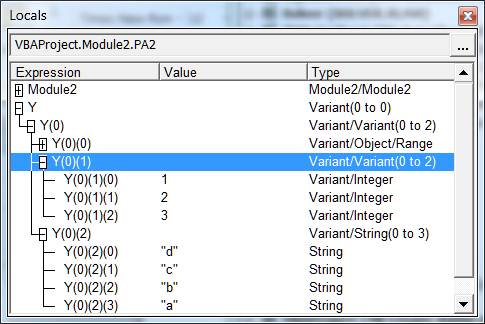# The ParamArray argument

The ParamArray argument makes it possible for a procedure (a function or a subroutine) to accept an arbitrary number of arguments, each of a possibly different type.  This enables the developer to write a function that will work with one or two or however many arguments that the user of the function passes to it.  The function in Code Sample 1 has a single ParamArray argument.  When used with a User Defined Function (UDF), it works as an Excel function.Figure 1

In VBA, a function with a ParamArray variable can be called as shown in Code Sample 1.

Option Explicit

Function MyMax(ParamArray X())

End Function

Sub testMyMax()

Debug.Print MyMax(Array(1, 2, 3))

Debug.Print MyMax(11, 222, 3333)

Debug.Print MyMax(Range("A1:A10"), Array(10, 20, 40))

Debug.Print MyMax(Array(1, 2, 3), 10, 11, 12)

Debug.Print MyMax(Split("d,c,b,a", ","))

End Sub

The example used here is a function (named MyMax) that returns the maximum value found in the argument passed to it.  As part of understanding the ParamArray argument, we will also fill out the MyMax function.

## Understanding the ParamArray variable

Each argument passed by the caller maps to an element in the ParamArray variable.  Consequently, the ParamArray variable will have as many elements as the arguments passed by the calling statement.

So, in the above example, the first call to MyMax will have X as a one element array.  That one element will contain an array, where the three elements of the array are the values 1, 2, and 3, respectively.  Since X is an array and the first element of X is an array, 2 indexes are required to access each value, e.g., X(0)(0) – see Figure 1, which shows the Visual Basic Editor’s Locals Window while executing code in the MyMax function.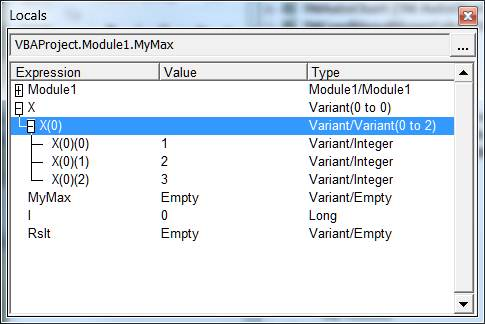The second call will cause X to have three elements, each of which contains one of the numbers passed to the function.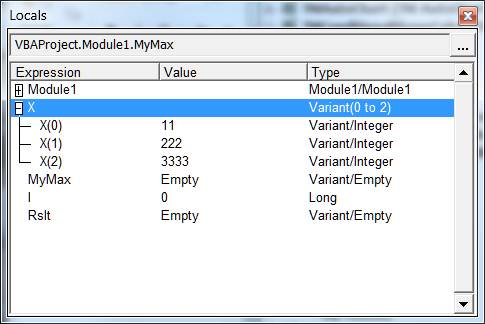Figure 3

For the third call, X will contain 2 elements.  The first will be a range object, the second an array.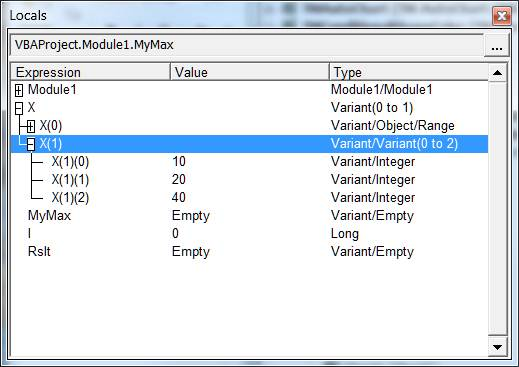Figure 4

## Working with the ParamArray variable

As we saw in the previous section the ParamArray variable contains 1 element for every argument actually passed to the function.  So, to work with the ParamArray we have to process each element of the array.  Since each element is of type variant, it becomes necessary to check the type of the element in it and process it appropriately.  Obviously, if the data type is something the developer does not expect, one can code a default way of process the data type or one can return an error.

For example, in the MyMax function, I opted to assume that an unknown data type will either have a default property or VBA itself will generate a fault.  So, the code checks if the type of the element is a range or an array and calls the appropriate function.  If it is neither, then it is either a simple data type (integer, long, single, double, Boolean, string) or an object other than a range or an array.  For an object the default property, if one exists, will be used.  If there is no default property, VBA will raise an error.

Function MyMax(ParamArray X())

Dim I As Long

For I = LBound(X) To UBound(X)

Dim Rslt

If TypeOf X(I) Is Range Then

Rslt = processRange(X(I))

If Rslt > MyMax Then MyMax = Rslt

ElseIf InStr(1, TypeName(X(I)), "(") > 0 Then 'array

Rslt = processArray(X(I))

If Rslt > MyMax Then MyMax = Rslt

ElseIf X(I) > MyMax Then  'if an object use the default value, if any

MyMax = X(I)

End If

Next I

End Function

There’s one thing that the reader may have already noticed.  Nowhere in the code is the value of MyMax initialized.  The first reference to it is in a comparison operation!  One way to initialize it is to set it to the smallest value possible.  Then, the first comparison will adjust it correctly.  Unfortunately, since the ParamArray variable can be any data type, we don’t know what “smallest value possible” means.  It could be a number.  It could be a string.  Or, it could be something else altogether!

A way to handle this uncertainty is to initialize MyMax to the value in the first element of the ParamArray variable.  But, of course, the first element itself may be an object (such as a range) or a structured data type (such as an array).  So, a simple MyMax=X(LBound(X)) is likely to fail.  Just like the check for the type of each element before processing it (Code Sample 2), first check the type of the first element before processing it.  Rather than add the code to the main routine, delegate the responsibility to a function and keep the main code simpler.

Function MyMax(ParamArray X())

MyMax = FirstVal(X(LBound(X)))

Dim I As Long

For I = LBound(X) To UBound(X)

Dim Rslt

If TypeOf X(I) Is Range Then

Rslt = processRange(X(I))

If Rslt > MyMax Then MyMax = Rslt

ElseIf InStr(1, TypeName(X(I)), "(") > 0 Then 'array

Rslt = processArray(X(I))

If Rslt > MyMax Then MyMax = Rslt

ElseIf X(I) > MyMax Then  'if an object use the default value, if any

MyMax = X(I)

End If

Next I

End Function

Code Sample 3

Since each of the elements of X is of type variant, the functions that process those elements must declare their sole argument as a variant.

The function FirstVal identifies the data type of its argument and returns the first or only element in it.  The code assumes that in the case of an array it is a single dimension array.

Option Explicit

Function FirstVal(X)

If TypeOf X Is Range Then

FirstVal = X.Cells(1).Value

ElseIf InStr(1, TypeName(X), "(") > 0 Then 'array

FirstVal = X(LBound(X)) 'assume 1D array

Else

FirstVal = X

End If

End Function

Code Sample 4

The processRange function steps through every cell in the range and returns the largest value.  Even though we know that it will only process a range, we cannot declare the argument as type Range.  This is because the type of the variable in the main routine is type variant.

Function processRange(X)

'X should be a range but cannot be declared as such

Dim Rslt

Rslt = X.Cells(1)

Dim aCell As Range

For Each aCell In X.Cells

If aCell.Value > Rslt Then Rslt = aCell.Value

Next aCell

processRange = Rslt

End Function

Code Sample 5

The next routine, processArray, assumes that its argument is a 1D array.  However, it doesn’t actually process the elements of the array.  Since each element of an array of type variant can itself contain an array or an object such as a Range, it calls MyMax recursively to process the array element.

Function processArray(X)

'X should be an array but cannot be declared as such

'Function assumes X is a 1D array

Dim Rslt

Rslt = MyMax(X(LBound(X)))

Dim I As Long

For I = LBound(X) + 1 To UBound(X)

Dim ThisRslt: ThisRslt = MyMax(X(I))

If ThisRslt > Rslt Then Rslt = ThisRslt

Next I

processArray = Rslt

End Function

Code Sample 6

## The ParamArray variable and other data types

So far, we have worked with a range, a single dimension array, and individual elements passed to the ParamArray variable.  These are the common data types encountered in the context of a user defined function or UDF.  The other common data types that one may want to consider are a 2D array, a Collection object, and a Dictionary object.  Each of them would require additional processing code.  Dealing with these objects would have distracted from understanding the ParamArray variable.  This section adds the code to process a 2D array.

Process the Collection and the Dictionary object similar to a 1D array.  Step through each element and recursively call the MyMax function with the element as the argument.

To handle a 2D array, we modify the processArray function so that it calls one of 2 different functions, process1DArr or process2DArr depending on the kind of array being processed.  Because the above is modularized, we only modify the processArray routine and don’t really need to retest any of the other data type routines.

Option Explicit

Function NbrDim(X)

Dim DimIdx As Integer

On Error GoTo XIT

Do

DimIdx = DimIdx + 1

Dim Temp: Temp = UBound(X, DimIdx)

Loop

XIT:

NbrDim = DimIdx - 1

End Function

Function process1DArr(X)

'Function assumes X is a 1D array

Dim Rslt

Rslt = MyMax(X(LBound(X)))

Dim I As Long

For I = LBound(X) + 1 To UBound(X)

Dim ThisRslt: ThisRslt = MyMax(X(I))

If ThisRslt > Rslt Then Rslt = ThisRslt

Next I

process1DArr = Rslt

End Function

Function process2DArr(X)

'Function assumes X is a 2D array

Dim Rslt

Rslt = MyMax(X(LBound(X), LBound(X, 2)))

Dim I As Long

For I = LBound(X) To UBound(X)

Dim J As Long

For J = LBound(X, 2) To UBound(X, 2)

Dim ThisRslt: ThisRslt = MyMax(X(I, J))

If ThisRslt > Rslt Then Rslt = ThisRslt

Next J

Next I

process2DArr = Rslt

End Function

Function processArray(X)

'X should be an array but cannot be declared as such

'Function assumes X is a 1D or 2D array

Select Case NbrDim(X)

Case 1: processArray = process1DArr(X)

Case 2: processArray = process2DArr(X)

Case Else:

End Select

End Function

Code Sample 7

## ParamArray together with other arguments

In the example used in this note, the ParamArray variable was the only argument.  That doesn’t have to be the case.  However, since the ParamArray accommodates an arbitrary number of arguments as defined by the caller, it must be the last of the arguments.

Function MyLarge(X As Long, ParamArray Y())

End Function

Code Sample 8

Since ParamArray allows an arbitrary number of arguments, it becomes impossible to combine it with optional arguments.  The Visual Basic Editor will raise an error for an unacceptable use of a ParamArray variable.

## Passing a ParamArray variable to another procedure

This is one place where the implementation of ParamArray feels a bit convoluted, though it is technically sound.  It is also not a major issue since whenever a ParamArray variable element contains an array we will anyway recursively call the subroutine.  Nonetheless, it’s something to be aware of.

The issue arises when a subroutine, say PA1, with a ParamArray variable passes that variable to another subroutine, call it PA2, which, of course, has a ParamArray argument.  Now, suppose that when PA1 gets called its ParamArray variable has several elements.  When PA1 calls PA2, its variable (X) shows up in the 1st element of PA2’s ParamArray variable (Y).  So, PA2 has an additional level of nesting, as it were, to go through to get to the original arguments.  Confused?  An example should clarify the issue.

In Code Sample 7 the subroutine PA1 declares its single argument as ParamArray.  When called, it proceeds to call PA2, which also declares its single argument as a ParamArray.

Option Explicit

Sub PA2(ParamArray Y())

End Sub

Sub PA1(ParamArray X())

PA2 X()

End Sub

Sub callPA1()

PA1 ActiveCell.CurrentRegion, Array(1, 2, 3), _

Split("d,c,b,a", ",")

End Sub

When callPA1 calls PA1 with three arguments, each of the arguments becomes an element in the ParamArray X (Figure 4).  So, in this subroutine, X(2) refers to the array result of the Split function.  Thus, X(2)(0) is the first character or “d”.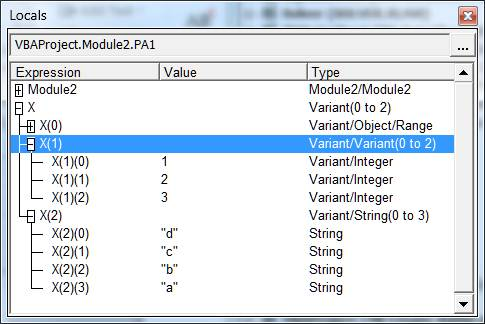Then, when PA1 calls PA2 it passes its ParamArray variable X to PA2’s ParamArray Y variable.  Since Y is a ParamArray variable, X – all of X – shows up in the first element of Y (Figure 5).  Practically, this adds an additional nesting level to the variables originally passed by callPA1.  So, Y(0) refers to the array X().  Then, Y(0)(0) refers to the first element of X and Y(0)(2) refers to the 3rd element of X, which is the array result of the Split function.  Thus, Y(0)(2)(0) refers to “d”, the 1st element of this array.